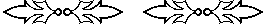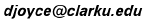## The Chinese algorithm for solving equations

David E. Joyce
Clark University

This algorithm will find a solution to a polynomial equation in one unknown one digit at a time. It has been known in China for a long time. The method was used for finding square roots and cube roots in particular, and for quadratic polynomials in general, about 2000 years ago as described in The Nine Chapters on the Mathematical Art (Jiuzhang Suanshu. Wang Xiaotong (fl. 625) used it to find roots of cubic polynomials, and later it was extended to solving higher degree equations and to equations with more than one unknown. See the page Mathematics in China at http://aleph0.clarku.edu/~djoyce/ma105/china.html for more details. In the West, it was developed much later by Horner, so the algorithm is also called “Horner’s method.”

The algorithm is best illustrated by example. We’ll consider two. First, a sample cubic equation, then a fifth degree equation used to find the fifth root of 2.

#### A sample cubic equation

Let’s try it on this example, a cubic equation:

x3 + 2x2 + 6x – 13258 = 0.

The constant here is 13258, so we need to find a cube near it. Now 103 is only 1000, too small, and 203 is only 8000, but closer. And 303 is 27000 which is too big. So, we expect the answer to be between 20 and 30. When you evaluate x3 + 2x2 + 6x – 13258 at x = 20, you find it’s negative, but it’s positive at x = 30. So we’re certain the solution is between 20 and 30. That means the first digit will be the 2 in 20.

Begin by writing, in columns here, the coefficients of the various powers of x. The large numbers, like 13258 will be grouped in triples of digits to make it easier to see the computations. (Putting digits in groups of 3 works well for this algorithm when the polynomial is cubic.)

```	x3	x2	   x1	      x0

1	2	   6	-13 258```
The following tableau shows the first set of computations. We can interpret this step with the help of modern symbolic algebra to see that it corresponds to setting x to y + 20 and deriving an equation for y. Under that interpretation, the tableau is a record of the computations. Note that the Chinese would have used a counting board, so that only the numbers would change, and at any given time there would be only four numbers on the board.
```	x3	 x2	   x1	      x0

1	 2	   6	-13 258
20		20	 440	  8 920
__      __       ___     ______
1	22       446	- 4 338
20		20	 840
__      __      ___
1	42      1286
20		20
__      __
1	62```
We now have the entries.
```	y3	y2	  y1	   y0

1	62	1286    -4 338```
This will have a solution less than 10. We need to find the next digit. Will it be 0,1, 2, ..., or 9? You can try various digits. Here's what happens when you try y = 5
```	y3	y2	  y1	    y0

1	62	1286	-4 338
5		 5	 335	 8 105
__      __      ____    ______
1	67	1621   whoops, it’s positive!```
So 5 doesn’t work; it’s too big. So is 4, and so is 3. But y = 2 gives a negative value. Therefore, y is between 2 and 3, so the next digit is 2. The following tableau shows the set of computations which corresponds to setting y to z + 2 and deriving an equation for z.
```	y3	y2	  y1	    y0

1	62	1286	-4 338
2		 2	 128	 2 828
__      __      ____    ______
1	64	1414	-1 510
2		 2	 132
__      __      ____
1	66	1546
2		 2
__      __
1	68```
We now have the entries.
```	z3	 z2	   z1	     z0

1	68	1546	-1 510```
The solution will be less than 1. What digit do we guess? To get a guess for the next digit, divide 1546, the coefficient of z, into 1510, the constant, to get about .9. As you can see, .9 will work.
```	z3	  z2	    z1	     z0

1	68  	1546   	-1 510
.9		  .9	  61.01	 1 446.309
__      ____    _______  _________
1	68.9	1607.01	   -55.691
.9		  .9	  62.82
__      ____    _______
1	69.8	1669.83
.9		  .9
__      ____
1	78.7```
We now have the entries.
```	w3	 w2	    w1	    w0

1	78.7	1669.83	-55.691```
The solution to this equation is now less than 0.1. In fact, it’s pretty close to 55.691/1669.83, about 0.033. The reason it should be close to that number is for w less than 1, the higher powers of w are pretty small, so they won’t contribute much at all. So, probably the first digit or two is correct. We could check them with the same algorithm, but we won’t. So we get the solution 22.93. Actually, it’s closer to 22.9375.

#### Finding the fifth root of 2

Take, for our second example, the problem of finding the 5th root of 2. To make it easier on decimals, let’s find the 5th root of 2 00000 00000 instead, which will be 100 times the 5th root of 2. Note that we’re grouping digits in groups of five in this example instead of three, since this is a 5th degree equation rather than a cubic. Now we need to solve the equation

x5 - 2 00000 00000 = 0.

Begin by writing, in columns here, the coefficients of the various powers of x.

```    x5    x4         x3          x2             x1                x0

1     0         0             0               0    -2 00000 00000
```

Now, we know the 5th root of 2 is between 1 and 2, so the the 5th root of 2 00000 00000 lies between 100 and 200, that is to say, the first digit is 1. The following tableau shows the first set of computations which corresponds to setting x to 100 + y and deriving an equation for y.

```    x5    x4         x3          x2             x1                x0

1     0         0             0               0    -2 00000 00000
100      100     10000     1 000 000     1 0000 0000     1 00000 00000
__  ____    ______     _________     ___________    ______________
1   100     10000     1 000 000     1 0000 0000    -1 00000 00000
100      100     20000     3 000 000     4 0000 0000
__  ____    ______     _________     ___________
1   200     30000     4 000 000     5 0000 0000
100      100     30000     6 000 000
__  ____    ______     _________
1   300     60000    10 000 000
100      100     40000
__  ____    ______
1   400    100000
100      100
__  ____
1   500
```

We now have the entries.

```    y5    y4        y3         y2              y1                y0

1   500    100000    10 000 000     5 0000 0000    -1 00000 00000
```

This will have a solution less than 100. We need to find its first digit. Will it be 10, 20, ..., or 90? Divide 500 000000 into 1 00000 00000, and you get 20. Actually, experience shows it’s going to be less than 20, so let’s try 10. The following tableau shows the next set of computations which corresponds to setting y to 10 + z and deriving an equation for z.

```    y5    y4        y3         y2              y1                y0

1   500    100000    10 000 000     5 0000 0000    -1 00000 00000
10        10      5100     1 051 000     1 1051 0000       61051 00000
__  ____    ______     _________     ___________    ______________
1   510    105100    11 051 000     6 1051 0000      -38949 00000
10        10      5200     1 103 000     1 2154 0000
__  ____    ______     _________     ___________
1   520    110300    12 154 000     7 3205 0000
10        10      5300     1 156 000
__  ____    ______     _________
1   530    115600    13 310 000
10        10      5400
__  ____    ______
1   540    121000
10        10
__  ____
1   550
```

We now have the entries.

```    z5    z4         z3        z2               z1                z0

1   550     121000   13 310 000     7 3205 0000      -38949 00000
```

The solution will be less than 10. What digit do we guess? Divide 732050000 into 389490000 to get about 5. So let’ try 5.

```    z5    z4         z3        z2               z1                z0

1   550     121000   13 310 000     7 3205 0000      -38949 00000
5          5       2775      618 875       6959 4375       40082 71875
__  ____    ______     _________     ___________    ______________
1   555     123775   13 918 875     8 0165 4375   whoops, it’s positive!
```

So 5 was too big. Let’s try 4 instead.

```    z5    z4         z3        z2               z1                z0

1   550     121000   13 310 000     7 3205 0000      -38949 00000
4          4       2116      492 464       5520 9856       31490 39424
__  ____    ______     _________     ___________    ______________
1   554     123116   13 802 464     7 8725 9856       -7458 60576
4          4       2232      501 392       5721 5424
__  ____    ______     _________    ___________
1   558     125348   14 303 856     8 4447 5280
4          4       2248      510 384
__  ____    ______     _________
1   562     127596   14 814 240
4          4       2264
__  ____     ______
1   566     129860
4          4
__  ____
1   570
```

We now have the entries.

```    w5    w4         w3        w2

1   570     129860   14 814 240     8 4447 5280       -7458 60576
```

The solution to this equation is now less than one. In fact, it’s pretty close to 745860576 / 844475280 = 0.88322. The reason it should be close to that number is for w less than 1, the higher powers of w are pretty small, so they won’t contriubute too much. So, probably the first digit or two is correct. So we get, as the 5th root of 2 the number 1.1488. Actually, it’s closer to 1.14869835.

This is a pretty nice algorithm. It’s fairly tedius, but a lot better than guessing.

#### The bisection algorithm

Are there other algorithms for solving equations? Yes. One is a continuous bisection method. For the bisection method, once you know where the root lies, for instance, we knew that a root of the polynomial x5 - 2 00000 00000 was between 100 and 200, then check the midpoint of the interval, 150 in our example. If the midpoint is not a root, which it usually isn’t, then the sign of the value of the polynomial will indicate whether the root is in the first half of the interval or the second half of the interval. For the example polynomial, x5 - 2 00000 00000, the value is positive when x is 100, but negative when x is 150 or 200, so a root lies between 100 and 150. Then 125 is tested, and so forth.

The Chinese algorithm is pretty much the same thing as the bisection algorithm, but the interval is divided into ten parts instead of into two parts. For human computation, division into ten parts is better, but for computers, division into two parts is slightly faster.

There are yet other algorithms to find roots of functions and solve equations. The bisection method works fine for any continuous functions, but for most of them, the differentiable functions, Newton’s method, which relies on calculus, is significantly faster.1999, 2002, 2004

Back to course page
David E. Joyce